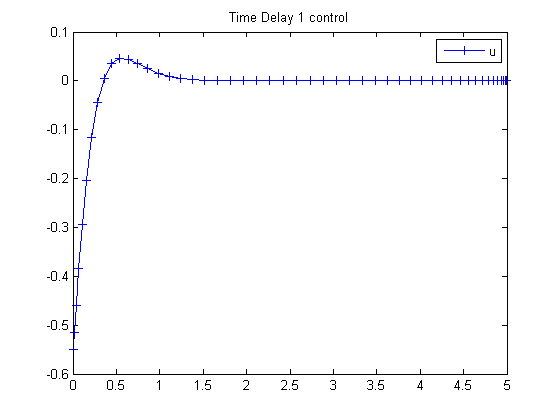113  Time Delay 1

ITERATIVE DYNAMIC PROGRAMMING, REIN LUUS

8.3.1 Example 1

CHAPMAN & HALL/CRC Monographs and Surveys in Pure and Applied Mathematics

Linear time-delay system used for optimal control studies by Chan and Perkins

113.1  Problem Formulation

Find u over t in [0; 5 ] to minimize

 J = x3(tF)

subject to:

 dx1 dt
= x2
 dx2 dt
= −10*x1−5*x2−2*x1(ttau)−x2(ttau)+u
 dx3 dt
= 0.5*(10*x12+x22+u2
 tau = 0.25

The initial condition are:

 x(t<=0) = [1  1  0]
 −inf <= u <= inf

Reference: 

113.2  Problem setup

toms t
p1 = tomPhase('p1', t, 0, 5, 50);
setPhase(p1);

tomStates x1 x2 x3
tomControls u

% Initial guess
x0 = {icollocate({x1 == 1
x2 == 1; x3 == 0})
collocate(u == 0)};

% Boundary constraints
cbnd = initial({x1 == 1; x2 == 1; x3 == 0});

% Expressions for x1(t-tau) and x2(t-tau)
tau = 0.25;
x1delayed = ifThenElse(t<tau, 1, subs(x1,t,t-tau));
x2delayed = ifThenElse(t<tau, 1, subs(x2,t,t-tau));

% ODEs and path constraints
ceq = collocate({dot(x1) == x2
dot(x2) == -10*x1 - 5*x2 - 2*x1delayed - x2delayed + u
dot(x3) == 0.5*(10*x1.^2+x2.^2+u.^2)});

% Objective
objective = final(x3);

113.3  Solve the problem

options = struct;
options.name = 'Time Delay 1';
solution = ezsolve(objective, {cbnd, ceq}, x0, options);
t  = subs(collocate(t),solution);
u  = subs(collocate(u),solution);
Problem type appears to be: lpcon
Starting numeric solver
===== * * * =================================================================== * * *
TOMLAB - Tomlab Optimization Inc. Development license  999001. Valid to 2011-02-05
=====================================================================================
Problem: ---  1: Time Delay 1                   f_k       2.525970860473679000
sum(|constr|)      0.000000011182005725
f(x_k) + sum(|constr|)      2.525970871655684600
f(x_0)      0.000000000000000000

Solver: snopt.  EXIT=0.  INFORM=1.
SNOPT 7.2-5 NLP code
Optimality conditions satisfied

FuncEv    1 ConstrEv   70 ConJacEv   70 Iter   49 MinorIter  205
CPU time: 0.671875 sec. Elapsed time: 0.687000 sec.

113.4  Plot result

figure(1)
plot(t,u,'+-');
legend('u');
title('Time Delay 1 control');